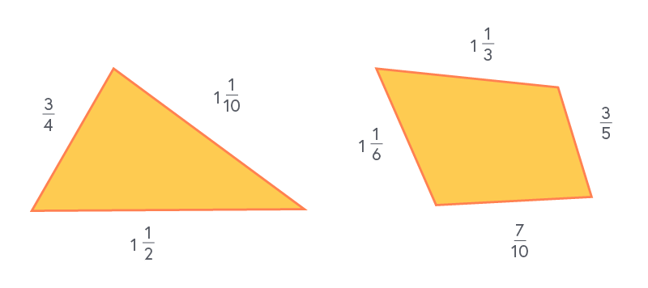## Want to keep learning?

This content is taken from the National STEM Learning Centre's online course, Maths Subject Knowledge: Fractions, Decimals, and Percentages. Join the course to learn more.
4.10

# Working with three or more fractions

In this step we work through two further problems where three or more fractions are added together.

## Example

Which of the two shapes below has the largest perimeter? The units for each side are the same and the drawing is not to scale.### Triangle perimeter

First, convert $$1\frac{1}{10}$$ to $$\frac{11}{10}$$, and convert $$1\frac{1}{2}$$ to $$\frac{3}{2}$$, so we are not working with mixed numbers.

The perimeter is equal to $$\frac{3}{4} + \frac{11}{10} + \frac{3}{2}$$.

To add these fractions, we look for the lowest common denominator across all three fractions, which is 20.

$$Perimeter_{T} = \frac{15}{20} + \frac{22}{20} + \frac{30}{20}$$ $$= \frac{67}{20}$$ $$= 3\frac{7}{20}$$

First, again to change from mixed numbers, convert $$1\frac{1}{6}$$ to $$\frac{7}{6}$$, and convert $$1\frac{1}{3}$$ to $$\frac{4}{3}$$.

The perimeter is the sum of all sides, which is $$\frac{7}{6} + \frac{4}{3} + \frac{3}{5} + \frac{7}{10}$$.

We look for the lowest common denominator for all four fractions, which is 30.

$$Perimeter_{Q} = \frac{35}{30} + \frac{40}{30} + \frac{18}{30} + \frac{21}{30}$$ $$= \frac{114}{30}$$ $$= 3\frac{24}{30}$$ $$= 3\frac{4}{5}$$

So both are ‘3 and something’. We need to compare the fractional parts of the number. To do this, we again find the lowest common denominator:

$$\frac{7}{20} = \frac{7}{20}$$ and $$\frac{4}{5} = \frac{16}{20}$$

$$3\frac{4}{5}$$ is bigger than $$3\frac{7}{20}$$, so the quadrilateral has the larger perimeter.

Next week we’ll be asking you to create and complete calculations with three or more fractions and different operators.